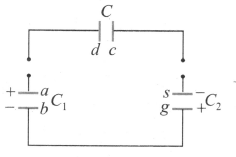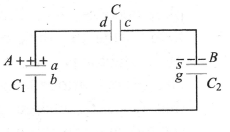Redistribution of charges and concept of common potential
Question

# Two capacitors A and B with capacities ${C}_{1} and {C}_{2}$ are charged to potential difference of ${V}_{1} and {V}_{2},$respectively. The plates of the capacitors are connected as shown in the figure. The upper plate of A is positive and that of B is negative. An uncharged capacitor of capacitance C and falls on the free ends to complete circuit, thenModerate
Solution

## Charge on positive plate of A is ${C}_{1}{V}_{1}.$ Charge on negative plate of B is  $-{C}_{2}{V}_{2}.$ When d plate of capacitor C is connected with the plate of A, then the total charge of (d, a) plate system will be ${C}_{1}{V}_{1}$ (conservation of charge).Get Instant Solutions